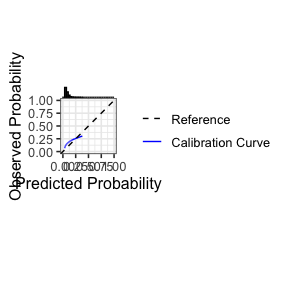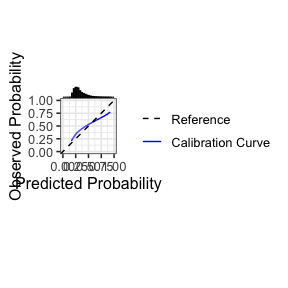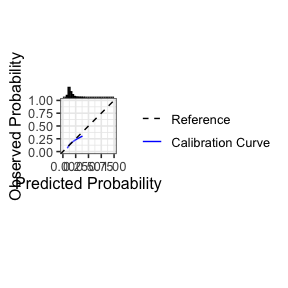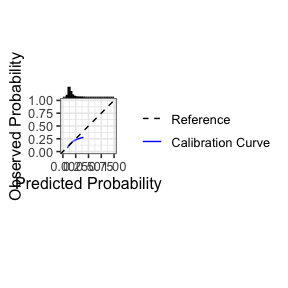# Preamble

The predRupdate package includes a set of functions to aid in the validation of a clinical prediction model (CPM) on a given dataset, and to apply various model updating and aggregation methods. This vignette aims to overview, through examples, some common workflows of using the predRupdate package. For a technical vignette describing the methods underpinning the package, please see vignette("predRupdate_technical").

The package is focused on the situation where there is an existing CPM (or multiple CPMs) that has been developed (e.g., a model published in the literature), and one wishes to apply this model to a new dataset. We foresee at least three use-cases: (1) where one wishes to validate the existing CPM on the new data to estimate the model’s predictive performance, i.e., external validation; (2) where one wishes to apply model updating methods to the existing CPM to ‘tailor’ it to the new dataset; and (3) where there are multiple existing CPMs, and one wishes to apply model aggregation (meta-modelling) methods to pool these models into a single model for the new dataset. We therefore give three examples below for each of these use cases.

# Data

The data, called SYNPM, used throughout this vignette are available within the predRupdate package. See “?SYNPM” for details of these data. In short, the data and models included in SYNPM are synthetic, but for the purposes of this vignette, we imagine that one is interested in predicting someone’s risk of mortality after surgery. Data are available on 20000 people, which records each individuals age, gender, smoking status, diabetes status, and Creatine value at the time of surgery. The data includes the outcomes of “ETime” representing the time (months) between surgery and either death or end-of-follow-up (5 months), whichever occurred first. The variable “Status” indicates if the patient died (1) or was right-censored (0), and Y denotes a binary variable indicating if the patient died within 1 month.

# Example 1: validating an existing prediction model on new data

In this first example, we take a situation where a CPM has previously been developed (in another dataset) to predict the risk of mortality within 1 month of surgery, and we wish to validate this model in our dataset to test the predictive performance (e.g., an external validation study).

The existing model was a logistic regression model, with the following predictor variables and coefficients (log-odds ratios):

Table of coefficients for the existing logistic regression prediction model
Coefficient
Intercept -3.995
Age 0.012
SexM 0.267
Smoking_Status 0.751
Diabetes 0.523
Creatine 0.578

The first step in using predRupdate to validate this model is to input the model information. We start by creating a data.frame of the model coefficients, with the columns being the predictor variable names. This information is then passed into the pred_input_info() function to input the information about the existing model. See pred_input_info() for details.

# create a data.frame of the model coefficients, with columns being variables
coefs_table <- data.frame("Intercept" = -3.995, #the intercept needs to be named exactly as given here
"Age" = 0.012,
"SexM" = 0.267,
"Smoking_Status" = 0.751,
"Diabetes" = 0.523,
"Creatine" = 0.578)

#pass this into pred_input_info()
Existing_Logistic_Model <- pred_input_info(model_type = "logistic",
model_info = coefs_table)
summary(Existing_Logistic_Model)
#> Information about 1 existing model(s) of type 'logistic'
#>
#> Model Coefficients
#> =================================
#>   Intercept   Age  SexM Smoking_Status Diabetes Creatine
#> 1    -3.995 0.012 0.267          0.751    0.523    0.578
#>
#> Model Functional Form
#> =================================
#> Age + SexM + Smoking_Status + Diabetes + Creatine

Next we wish to apply this model to our dataset to calculate the predicted risks for each individual, and then compare these predictions with the observed outcomes to calculate predictive performance. This can all be achieved with the pred_validate() function, as follows:

validation_results <- pred_validate(x = Existing_Logistic_Model,
new_data = SYNPM$ValidationData, binary_outcome = "Y")summary(validation_results) #use summary() to obtain a tidy output summary of the model performance #> Calibration Measures #> --------------------------------- #> Estimate Std. Err Lower 95% Confidence Interval #> Calibration-in-the-large 0.7076 0.0206 0.6673 #> Calibration Slope 0.6496 0.0463 0.5588 #> Upper 95% Confidence Interval #> Calibration-in-the-large 0.7479 #> Calibration Slope 0.7403 #> #> Also examine the calibration plot, if produced. #> #> Discrimination Measures #> --------------------------------- #> Estimate Std. Err Lower 95% Confidence Interval #> AUC 0.5816 0.0057 0.5703 #> Upper 95% Confidence Interval #> AUC 0.5928 #> #> #> Overall Performance Measures #> --------------------------------- #> Cox-Snell R-squared: -0.0446 #> Nagelkerke R-squared: -0.0801 #> Brier Score: 0.1246 #> #> Also examine the histogram of predicted risks. This produces a flexible calibration plot, along with outputting various metrics of model calibration (e.g., calibration intercept and slope), discrimination (e.g., area under the ROC curve) and overall performance (e.g., R-squared). We can see that this model has poor calibration (calibration plot deviating from the y=x line, with calibration intercept and slope significantly different from 0 and 1, respectively), and poor discrimination. One may wish to update this model to the new dataset - see Example 2 below. ## Survival analysis model The above example considered the validation of an existing CPM that was based on logistic regression. predRupdate also contains functionality to validate CPMs that are based on time-to-event (survival) models (e.g. a Cox proportional hazards model). In such a case, the baseline cumulative hazard of the model should also be specified, along with the regression coefficients. In many cases, the baseline cumulative hazard of an existing CPM will not be reported in “full”, but rather estimates of the baseline cumulative hazard will be given at set follow-up times. To use predRupdate, users should specify the baseline cumulative hazard at the times at which one wishes to make predictions (or validate/update the model). For example, suppose an existing CPM was developed using Cox proportional hazards to predict time-to-death after surgery, with the following predictor parameters (log hazard ratios): Table of coefficients for the existing time-to-event regression prediction model Coefficient Age 0.007 SexM 0.225 Smoking_Status 0.685 Diabetes 0.425 Creatine 0.587 and with the following baseline cumulative hazard reported at discrete times of months 1-5 post surgery: Table of baseline cumulative hazard time hazard 1 0.0230191 2 0.0475351 3 0.0720775 4 0.0969976 5 0.1209202 We can then use the pred_validate() function to validate this model at 1, 2, 3, 4 or 5 months follow-up in the new dataset. To achieve this, one follows a similar syntax to above for the logistic model. The main difference is that one needs to specify a time during follow-up that we’d like to validate the model against - this time must also be available in the baseline cumulative hazard provided. # create a data.frame of the model coefficients, with columns being variables coefs_table <- data.frame("Age" = 0.007, "SexM" = 0.225, "Smoking_Status" = 0.685, "Diabetes" = 0.425, "Creatine" = 0.587) #pass this into pred_input_info() Existing_TTE_Model <- pred_input_info(model_type = "survival", model_info = coefs_table, cum_hazard = BH_table) #where BH_table is the baseline hazard above #now validate against the time-to-event outcomes in the new dataset: validation_results <- pred_validate(x = Existing_TTE_Model, new_data = SYNPM$ValidationData,
survival_time = "ETime",
event_indicator = "Status",
time_horizon = 5)summary(validation_results)
#> Calibration Measures
#> ---------------------------------
#>                         Estimate Std. Err Lower 95% Confidence Interval
#> Observed:Expected Ratio   1.2211   0.0113                        1.1944
#> Calibration Slope         0.7129   0.0281                        0.6580
#>                         Upper 95% Confidence Interval
#> Observed:Expected Ratio                        1.2484
#> Calibration Slope                              0.7679
#>
#>  Also examine the calibration plot, if produced.
#>
#> Discrimination Measures
#> ---------------------------------
#>           Estimate Std. Err Lower 95% Confidence Interval
#> Harrell C   0.5789   0.0032                        0.5726
#>           Upper 95% Confidence Interval
#> Harrell C                        0.5852
#>
#>  Also examine the histogram of predicted risks.

Here, we see that the existing model under-predicts the mean risk at 5-months (observed:Expected Ratio greater than 1), and there is evidence of over-fitting (both from the calibration plot and the calibration slope being significantly different from 1). The model also has poor discrimination (Harrell C).

### Specifying the baseline cumulative hazard

When validating an existing time-to-event model, the baseline cumulative hazard of the existing CPM should be reported (e.g., from the original model publication). In some cases, this might be reported at discrete follow-up times (like in the above example). Alternatively, the entire baseline cumulative hazard curve may be presented, or indeed a parametric form of the baseline cumulative hazard may be provided. In those situations, one should extract (from the plot) or calculate (from the parametric form) the baseline cumulative hazard at multiple follow-up times of interest (i.e., the follow-up times at which one wishes to validate the model against).

However, in some cases, the baseline cumulative hazard of the existing time-to-event CPM may not be reported. In such a situation, one can still use predRupdate to validate such a model. However, only a limited number of metrics will be produced (i.e., only those metrics that require the linear predictor, not absolute risk predictions at a given follow-up time). Specifically, the observed:expected ratio and the calibration plot will not be produced if the baseline cumulative hazard is not provided.

For example, suppose the baseline cumulative hazard for the above model was not available. We could validate this model using predRupdate as follows:

# create a data.frame of the model coefficients, with columns being variables
coefs_table <- data.frame("Age" = 0.007,
"SexM" = 0.225,
"Smoking_Status" = 0.685,
"Diabetes" = 0.425,
"Creatine" = 0.587)

#pass this into pred_input_info()
Existing_TTE_Model <- pred_input_info(model_type = "survival",
model_info = coefs_table,
cum_hazard = NULL) #leave as NULL if the baseline not available

#now validate against the time-to-event outcomes in the new dataset:
validation_results <- pred_validate(x = Existing_TTE_Model,
new_data = SYNPM$ValidationData, survival_time = "ETime", event_indicator = "Status", time_horizon = 5) #> Warning: Predicted risks are not available for some models: limited calibration #> metrics returned and no calibration plot produced summary(validation_results) #> Calibration Measures #> --------------------------------- #> Estimate Std. Err Lower 95% Confidence Interval #> Observed:Expected Ratio NA NA NA #> Calibration Slope 0.7129 0.0281 0.658 #> Upper 95% Confidence Interval #> Observed:Expected Ratio NA #> Calibration Slope 0.7679 #> #> Also examine the calibration plot, if produced. #> #> Discrimination Measures #> --------------------------------- #> Estimate Std. Err Lower 95% Confidence Interval #> Harrell C 0.5789 0.0032 0.5726 #> Upper 95% Confidence Interval #> Harrell C 0.5852 #> #> Also examine the histogram of predicted risks. Here, we see that no calibration plot is produced, and the observed:expected ratio is NA. A warning message is given to highlight this. # Example 2: model updating on new data In the validation of an existing logistic regression model in Example 1 above, we found that the existing model was miscalibrated in the new data. One strategy to handle this is to apply a range of model updating methods; see vignette("predRupdate_technical") for a technical discussion of these methods. To illustrate how to achieve this with predRupdate, we here choose to apply model re-calibration to the existing logistic regression model shown in Example 1 above. Within predRupdate, we use pred_update(): # create a data.frame of the model coefficients, with columns being variables coefs_table <- data.frame("Intercept" = -3.995, "Age" = 0.012, "SexM" = 0.267, "Smoking_Status" = 0.751, "Diabetes" = 0.523, "Creatine" = 0.578) #pass this into pred_input_info() Existing_Logistic_Model <- pred_input_info(model_type = "logistic", model_info = coefs_table) #apply the pred_update function to update the model to the new dataset: Updated_model <- pred_update(Existing_Logistic_Model, update_type = "recalibration", new_data = SYNPM$ValidationData,
binary_outcome = "Y")

summary(Updated_model)
#> Original model was updated with type recalibration
#> The model updating results are as follows:
#>               Estimate Std. Error
#> (Intercept) -0.1579827 0.11713282
#> Slope        0.6495556 0.04629572
#>
#> Updated Model Coefficients
#> =================================
#>   Intercept         Age      SexM Smoking_Status  Diabetes  Creatine
#> 1 -2.752957 0.007794668 0.1734314      0.4878163 0.3397176 0.3754432
#>
#> Model Functional Form
#> =================================
#> Age + SexM + Smoking_Status + Diabetes + Creatine

One could then validate this updated model using pred_validate(), but given we have updated the model in the new data, then in-practice any such performance estimates would need to be adjusted for in-sample optimism (e.g., using cross-validation or bootstrap internal validation):

summary(pred_validate(Updated_model,
new_data = SYNPM$ValidationData, binary_outcome = "Y"))#> Calibration Measures #> --------------------------------- #> Estimate Std. Err Lower 95% Confidence Interval #> Calibration-in-the-large 0 0.0204 -0.0400 #> Calibration Slope 1 0.0713 0.8603 #> Upper 95% Confidence Interval #> Calibration-in-the-large 0.0400 #> Calibration Slope 1.1397 #> #> Also examine the calibration plot, if produced. #> #> Discrimination Measures #> --------------------------------- #> Estimate Std. Err Lower 95% Confidence Interval #> AUC 0.5816 0.0057 0.5703 #> Upper 95% Confidence Interval #> AUC 0.5928 #> #> #> Overall Performance Measures #> --------------------------------- #> Cox-Snell R-squared: 0.0095 #> Nagelkerke R-squared: 0.0171 #> Brier Score: 0.1203 #> #> Also examine the histogram of predicted risks. Similar functionality is available for time-to-event models, using a similar syntax. # Example 3: model aggregation on new data Sometimes, there might be multiple existing CPMs available for the same prediction task (e.g., existing models developed across different countries, each aiming to predict the same outcome). Here, model aggregation methods can be used to pool these existing CPMs into a single model in the new data; see vignette("predRupdate_technical") for a technical discussion of these methods. To implement these methods within predRupdate, we first need to input the information about the multiple existing models into pred_input_info(). Each row of the model_info parameter should be the coefficients of an existing CPM; any parameter that is not included in a given CPM should have value NA, as shown below: coefs_table <- data.frame(rbind(c("Intercept" = -3.995, "Age" = 0.012, "SexM" = 0.267, "Smoking_Status" = 0.751, "Diabetes" = 0.523, "Creatine" = 0.578), c("Intercept" = -2.282, "Age" = NA, "SexM" = 0.223, "Smoking_Status" = 0.528, "Diabetes" = 0.200, "Creatine" = 0.434), c("Intercept" = -3.013, "Age" = NA, "SexM" = NA, "Smoking_Status" = 0.565, "Diabetes" = -0.122, "Creatine" = 0.731))) multiple_mods <- pred_input_info(model_type = "logistic", model_info = coefs_table) summary(multiple_mods) #> Information about 3 existing model(s) of type 'logistic' #> #> Model Coefficients #> ================================= #> [] #> Intercept Age SexM Smoking_Status Diabetes Creatine #> 1 -3.995 0.012 0.267 0.751 0.523 0.578 #> #> [] #> Intercept SexM Smoking_Status Diabetes Creatine #> 2 -2.282 0.223 0.528 0.2 0.434 #> #> [] #> Intercept Smoking_Status Diabetes Creatine #> 3 -3.013 0.565 -0.122 0.731 #> #> #> Model Functional Form #> ================================= #> Model 1: Age + SexM + Smoking_Status + Diabetes + Creatine #> Model 2: SexM + Smoking_Status + Diabetes + Creatine #> Model 3: Smoking_Status + Diabetes + Creatine We can then use pred_stacked_regression() to apply stacked regression to aggregate these models into a single model for the new dataset: SR <- pred_stacked_regression(x = multiple_mods, new_data = SYNPM$ValidationData,
binary_outcome = "Y")
summary(SR)
#> Existing models aggregated using stacked regression
#> The model stacked regression weights are as follows:
#> (Intercept)         LP1         LP2         LP3
#>  0.02072515  0.48150208  0.12920227  0.16559034
#>
#> Updated Model Coefficients
#> =================================
#>   Intercept         Age      SexM Smoking_Status Diabetes  Creatine
#> 1 -2.696639 0.005778025 0.1573732      0.5233854 0.257464 0.4554285
#>
#> Model Functional Form
#> =================================
#> Age + SexM + Smoking_Status + Diabetes + Creatine

One could then validate this updated model using pred_validate(), but given we have updated the model in the new data, then in-practice any such performance estimates would need to be adjusted for in-sample optimism (e.g., using cross-validation or bootstrap internal validation):

summary(pred_validate(SR,
new_data = SYNPM$ValidationData, binary_outcome = "Y"))#> Calibration Measures #> --------------------------------- #> Estimate Std. Err Lower 95% Confidence Interval #> Calibration-in-the-large 0 0.0204 -0.0400 #> Calibration Slope 1 0.0702 0.8623 #> Upper 95% Confidence Interval #> Calibration-in-the-large 0.0400 #> Calibration Slope 1.1377 #> #> Also examine the calibration plot, if produced. #> #> Discrimination Measures #> --------------------------------- #> Estimate Std. Err Lower 95% Confidence Interval #> AUC 0.583 0.0058 0.5717 #> Upper 95% Confidence Interval #> AUC 0.5943 #> #> #> Overall Performance Measures #> --------------------------------- #> Cox-Snell R-squared: 0.0098 #> Nagelkerke R-squared: 0.0175 #> Brier Score: 0.1203 #> #> Also examine the histogram of predicted risks. Similar functionality is available for time-to-event models. # A note on structure of new_data As shown above, the workflow within predRupdate is broadly in two main stages: (i) input information about the existing CPM(s) using pred_input_info(), and then (ii) make predictions, perform validation, perform model updating or conduct model aggregation methods using pred_predict() pred_validate(), pred_update() or pred_stacked_regression(), respectively. Stage (i) requires specification of the ‘model_info’ parameter (which contains the information of the model parameters), and stage (ii) requires specification of the ‘new_data’ on which one wishes to apply the existing CPM(s). It is a requirement that there is consistency between the parameter/coefficient names given in the ‘model_info’ parameter of pred_input_info() and the names of variables in “new_data” of pred_predict() pred_validate(), pred_update() or pred_stacked_regression(). Specifically, each coefficient named in ‘model_info’ should also be a column of ‘new_data’. For example, the following results in an error, because the smoking variable passed through model_info in pred_input_info() does not match a name of the smoking variable in ‘new_data’ passed to pred_predict(). # create a data.frame of the model coefficients, with columns being variables coefs_table <- data.frame("Intercept" = -3.995, "Smoking" = 0.751) #pass this into pred_input_info() Existing_Logistic_Model <- pred_input_info(model_type = "logistic", model_info = coefs_table) try(pred_predict(Existing_Logistic_Model, new_data = SYNPM$ValidationData))
#> Error in map_newdata.predinfo_logistic(x = x, new_data = new_data, binary_outcome = binary_outcome,  :
#>   new_data does not contain some of the predictor variables for the model(s) specified within the pminfo object

names(SYNPM$ValidationData) #>  "Age" "SexM" "Smoking_Status" "Diabetes" #>  "Creatine" "ETime" "Status" "Y" Moreover, particular care should be given to any categorical/factor variables within new_data. For example, suppose we have the following new_data: new_df <- data.frame("Sex" = as.factor(c("M", "F", "M", "M", "F", "F", "M")), "Smoking_Status" = c(1, 0, 0, 1, 1, 0, 1)) Here, Sex is recorded as a factor variable with levels “M” and “F”. Before working with functions in predRupdate, one must first convert any factor variables to indicator/dummy variables. We provide dummy_vars() within predRupdate to assist with this. For example: coefs_table <- data.frame("Intercept" = -3.4, "Sex_M" = 0.306, "Smoking_Status" = 0.628) existing_Logistic_Model <- pred_input_info(model_type = "logistic", model_info = coefs_table) #if we try to use functions within predRupdate using new_df it will give an error as Sex is a factor variable: try(pred_predict(existing_Logistic_Model, new_data = new_df)) #> Error in map_newdata.predinfo_logistic(x = x, new_data = new_data, binary_outcome = binary_outcome, : #> 'new_data' contains factor variables - convert to dummy/indicator variables first #> dummy_vars() can help with this #we must first turn into dummy variables: new_df_indicatorvars <- dummy_vars(new_df) head(new_df_indicatorvars) #> Smoking_Status Sex_F Sex_M #> 1 1 0 1 #> 2 0 1 0 #> 3 0 0 1 #> 4 1 0 1 #> 5 1 1 0 #> 6 0 1 0 #and then pass to functions within predRupdate; e.g.: pred_predict(existing_Logistic_Model, new_data = new_df_indicatorvars) #>$LinearPredictor
#>  -2.466 -3.400 -3.094 -2.466 -2.772 -3.400 -2.466
#>
#> $PredictedRisk #>  0.07827635 0.03229546 0.04335543 0.07827635 0.05885613 0.03229546 0.07827635 #> #>$Outcomes
#> NULL